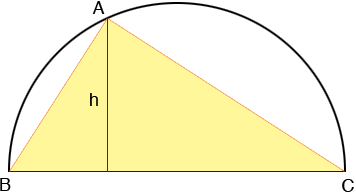SEARCH HOMEMath Central Quandaries & QueriesSubject: Limiting Cases in Geometry Name: Benneth Who are you: Student Consider a triangle inscribed in a semicircle with a radius of R. What are the possible perimeters for the triangle? And the areas?Hi Benneth,

I think you are to inscribe the triangle so that one side is the diameter of the semicircle.The area of the triangle is one-half the base (2R) times the height (h). If A is at B then the height is zero. How large can the height be?

For the perimeter use the fact that angle CAB is a right angle and apply Pythagoras theorem.

PennyMath Central is supported by the University of Regina and The Pacific Institute for the Mathematical Sciences.